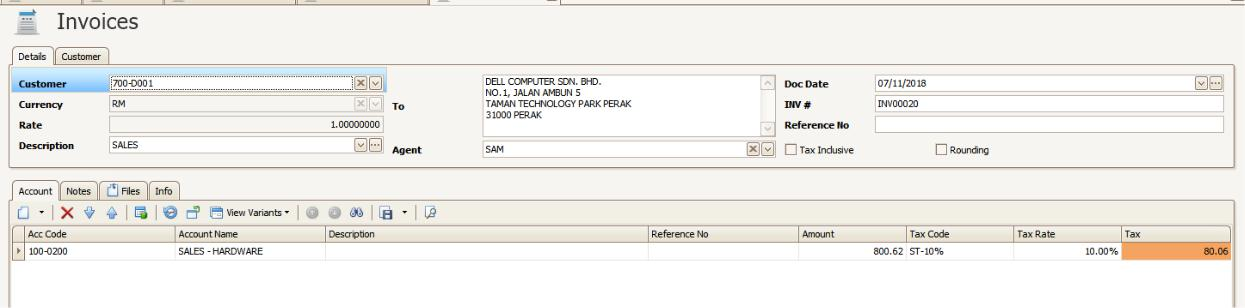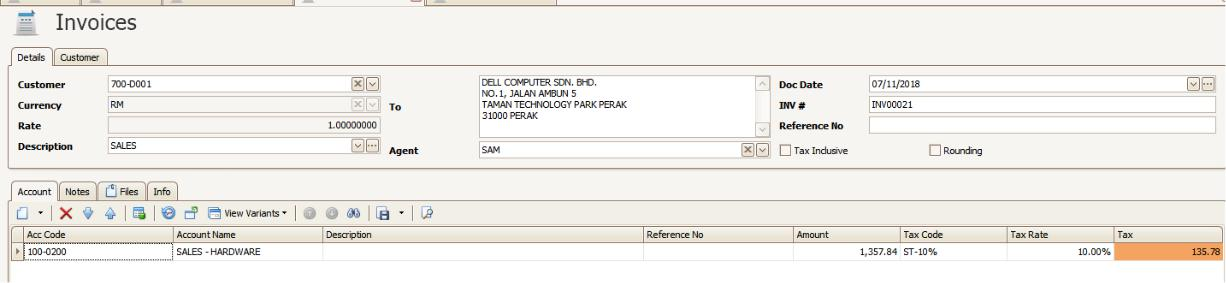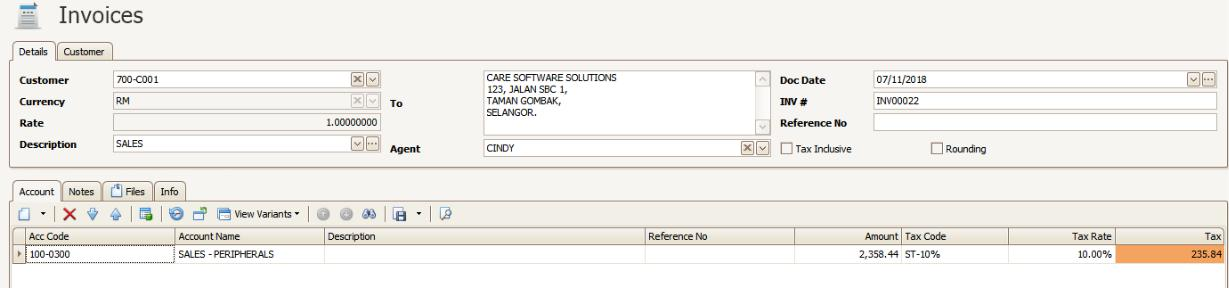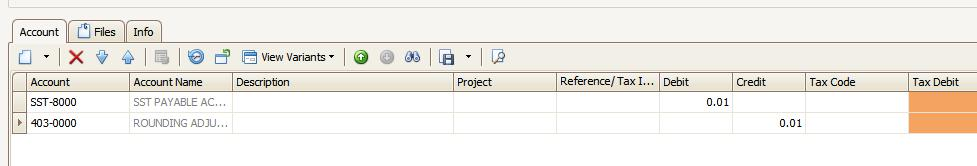SCENARIO:

In QnE Optimum that SST Tax Amount is total up by Document Tax Amount but for SST Online Submission that Tax Amount is Total Amount x 10%.

Example:

Create 1st Invoice > Amount = 800.62 > Tax Amount = 80.06.Create 2nd Invoice > Amount = 1357.84 > Tax Amount = 135.78Create 3rd Invoice > Amount = 2358.44 > Tax Amount = 235.84.Total Tax Amount on QnE Optimum = 80.06 + 135.78 +235.84

=451.68

=4516.90 x 10%

=451.69

so that have 1 cent different between system and SST Online Submissions.

SOLUTION:

On this situation, user need to do the adjustment on Journal Entries for this.

Example:

JV Date                                = Follow Tax Submisssion Date

DR- SST Payable Account    = 0.01

CR – Rounding Adjustment = 0.01